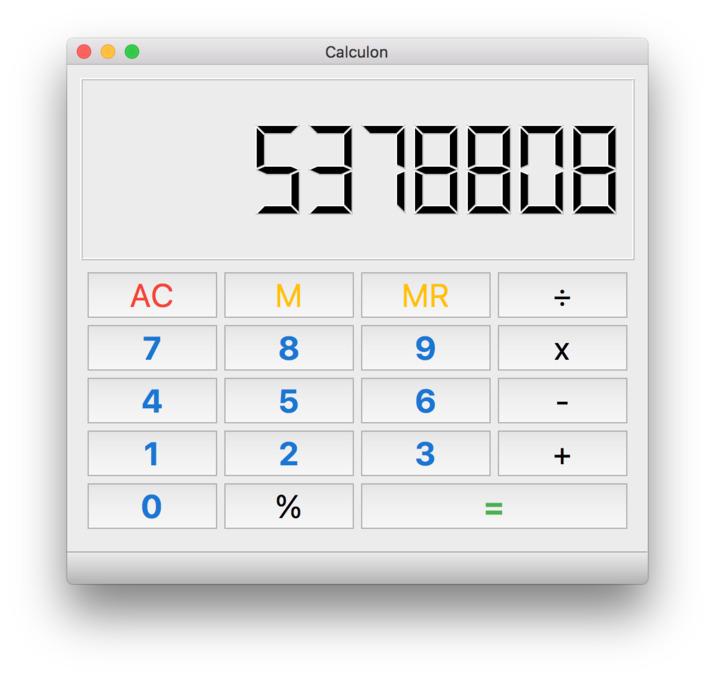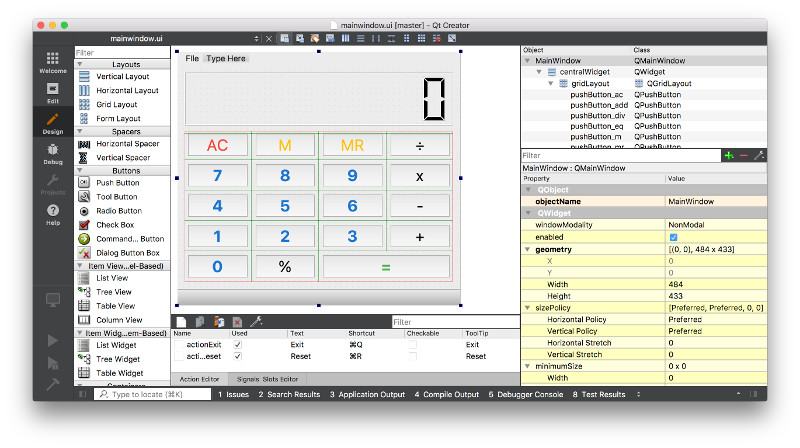# Calculon Writing a simple desktop calculator in Python

Calculators are one of the simplest desktop applications, found by default on every window system. Over time these have been extended to support scientific and programmer modes, but fundamentally they all work the same.

In this short write up we implement a working standard desktop calculator using PyQt. This implementation uses a 3-part logic — including a short stack, operator and state. Basic memory operations are also implemented.

While this is implemented for Qt, you could easily convert the logic to work in hardware with MicroPython or a Raspberry Pi.The full source code for Calculon is available in the 15 minute apps repository. You can download/clone to get a working copy, then install requirements using:

```pip3 install -r requirements.txt
```

You can then run Calculon with:

```python3 notepad.py
```

Read on for a walkthrough of how the code works.

## User interface

The user interface for Calculon was created in Qt Designer. The layout of the mainwindow uses a `QVBoxLayout` with the LCD display added to the top, and a `QGridLayout` to the bottom.

We use the grid layout is used to position all the buttons for the calculator. Each button takes a single space on the grid, except for the equals sign which is set to span two squares.Each button is defined with a keyboard shortcut to trigger a `.pressed` signal — e.g. `3` for the 3 key. The actions for each button are defined in code and connected to this signal.

If you want to edit the design in Qt Designer, remember to regenerate the `MainWindow.py` file using `pyuic5 mainwindow.ui -o MainWindow.py`.

## Actions

To make the buttons do something we need to connect them up to specific handlers. The connections defined are shown first below, and then the handlers covered in detail.

First we connect all the numeric buttons to the same handler. In Qt Designer we named all the buttons using a standard format, as `pushButton_nX` where `X` is the number. This makes it simple to iterate over each one and connect it up.

We use a function wrapper on the signal to send additional data with each trigger — in this case the number which was pressed.

```    for n in range(0, 10):
getattr(self, 'pushButton_n%s' % n).pressed.connect(lambda v=n: self.input_number(v))
```

The next block of signals to connect are for standard calculator operations, including add, multiply, subtraction and divide. Again these are hooked up to the same slot, and consist of a wrapped signal to transmit the operation (an specific Python `operator` type).

```    self.pushButton_add.pressed.connect(lambda: self.operation(operator.add))
self.pushButton_sub.pressed.connect(lambda: self.operation(operator.sub))
self.pushButton_mul.pressed.connect(lambda: self.operation(operator.mul))
self.pushButton_div.pressed.connect(lambda: self.operation(operator.truediv))  # operator.div for Python2.7
```

In addition to the numbers and operators, we have a number of custom behaviours to wire up — percentage (to convert the previously typed number to a percentage amount), equals, reset and memory actions.

```    self.pushButton_pc.pressed.connect(self.operation_pc)
self.pushButton_eq.pressed.connect(self.equals)

self.pushButton_ac.pressed.connect(self.reset)

self.pushButton_m.pressed.connect(self.memory_store)
self.pushButton_mr.pressed.connect(self.memory_recall)
```

Now the buttons and actions are wired up, we can implement the logic in the slot methods for handling these events.

### Want to build your own apps?

Then you might enjoy this book! Create Simple GUI Applications with Python & Qt is my guide to building cross-platform GUI applications with Python. Work step by step from displaying your first window to building fully functional desktop software.

## Operations

Calculator operations are handled using three components — the stack, the state and the current operation.

### The `stack`

The `stack` is a short memory store of maximum 2 elements, which holds the numeric values with which we're currently calculating. When the user starts entering a new number it is added to the end of the stack (which, if the stack is empty, is also the beginning). Each numeric press multiplies the current stack end value by 10, and adds the value pressed.

```def input_number(self, v):
if self.state == READY:
self.state = INPUT
self.stack[-1] = v
else:
self.stack[-1] = self.stack[-1] * 10 + v

self.display()
```

This has the effect of numbers filling from the right as expected, e.g.

Value pressed Calculation Stack
0
2 0 * 10 + 2 2
3 2 * 10 + 3 23
5 23 * 10 + 5 235

### The `state`

A `state` flag, to toggle between ready and input states. This affects the behaviour while entering numbers. In ready mode, the value entered is set direct onto the stack at the current position. In input mode the above shift+add logic is used.

This is required so it is possible to type over a result of a calculation, rather than have new numbers added to the result of the previous calculation.

```def input_number(self, v):
if self.state == READY:
self.state = INPUT
self.stack[-1] = v
else:
self.stack[-1] = self.stack[-1] * 10 + v

self.display()
```

You'll see switches between `READY` and `INPUT` states elsewhere in the code.

### The `current_op`

The `current_op` variable stores the currently active operation, which will be applied when the user presses equals. If an operation is already in progress, we first calculate the result of that operation, pushing the result onto the stack, and then apply the new one.

Starting a new operation also pushes `0` onto the stack, making it now length 2, and switches to `INPUT` mode. This ensures any subsequent number input will start from zero.

```def operation(self, op):
if self.current_op:  # Complete the current operation
self.equals()

self.stack.append(0)
self.state = INPUT
self.current_op = op
```

The operation handler for percentage calculation works a little differently. This instead operates directly on the current contents of the stack. Triggering the `operation_pc` takes the last value in the stack and divides it by 100.

```def operation_pc(self):
self.state = INPUT
self.stack[-1] *= 0.01
self.display()
```

## Equals

The core of the calculator is the handler which actually does the maths. All operations (with the exception of percentage) are handled by the `equals` handler, which is triggered either by pressing the equals key, Enter or another operation key while an op is in progress.

The equals handler takes the `current_op` and applies it to the values in the stack (2 values, unpacked using `*self.stack`) to get the result. The result is put back in the stack as a single value, and we return to a `READY` state. Errors (exceptions, e.g. for division by zero) are caught and an error message is displayed if neccessary.

```def equals(self):
# Support to allow '=' to repeat previous operation
# if no further input has been added.
if self.state == READY and self.last_operation:
s, self.current_op = self.last_operation
self.stack.append(s)

if self.current_op:
self.last_operation = self.stack[-1], self.current_op

try:
self.stack = [self.current_op(*self.stack)]
except Exception:
self.lcdNumber.display('Err')
self.stack = 
else:
self.current_op = None
self.display()
```

Support has also been added for repeating previous operations by pressing the equals key again. This is done by storing the value and operator when equals is triggered, and re-using them if equals is pressed again without leaving `READY` mode (no user input).

## Memory

Finally, we can define the handlers for the memory actions. For Calculon we've defined only two memory actions — store and recall. Store takes the current value from the LCD display, and copies it to `self.memory`. Recall takes the value in `self.memory` and puts in the final place on our stack.

```def memory_store(self):
self.memory = self.lcdNumber.value()

def memory_recall(self):
self.state = INPUT
self.stack[-1] = self.memory
self.display()
```

By setting the mode to INPUT and updating the display this behaviour is the same as for entering a number by hand.

## Future ideas

The current implementation of Calculon only supports basic math operations. Most GUI desktop calculators also include support for scientific (and sometimes programmer) modes, which add a number or alternative functions.

In Calculon you could define these additional operations as a set of `lambda`s, which each accept the two parameters to operate on.

Switching modes (e.g. between normal and scientific) on the calculator will be tricky with the current `QMainWindow`-based layout. You could rework the calculator layout in QtDesigner to use a `QWidget` base. Each view is just a widget, and switching modes can be performed by swapping out the central widget on your running main window.